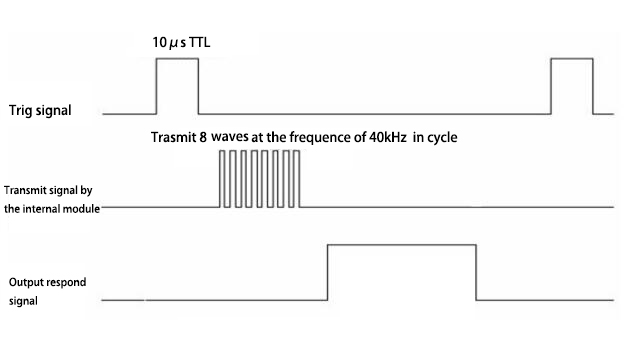HC-SR04

Introduction

HC-SR04 Ultrasonic Ranging Sensor module using ultrasonic to measure the distance. Compared to infrared sensor module, HC-SR04 is more accuracy and performant more stably, and HC-SR has longer detecting range, distance. The distance can be reach 5meters, besides, the measure angle is 45°, it’s convenient to measure the distance.

Look at the picture, there are two sensors, one emits the ultrasonic and the other one receives it when the ultrasonic is reflect by the obstacle, there are also two pins for emits and receiving the data from controller.Features

Power supply: 5V DC.

Effectual angle: <15°.

Ranging distance: 50px to 500 cm.

Resolution: 1 cm.

Ultrasonic Frequency: 40k Hz.

Note: the size of the object is better to be more than 0.5 m2, the surface is as possible as smooth, or it will influence the accuracy of measure data.

usage

The principle of the HC-SR04: it transmits a short ultrasonic pulse, receive the signal which reflected by the object, and converts it into a electric signal.

Here’s the sequence chart:First, we transmit a trig signal which is high lever signal at least 10 μs to the HC-SR04, then it transmits 8 square waves at the frequency of 40 kHz in cycle, it will detect if there’s the return signal by itself, when the square waves reflected by the object, HC-SR04 transmit a high lever signal, the time of the high lever is proportional to the distance, therefore, it’s easy to get the distance by account the high lever’s time and then divide it by 58. How do I get this?

Here’s the program of how to measure the distance by use of HC-SR04 equation? First of all, the speed of the voice is normally 340 m/s, the unit of time we get is μs, and the unit of distance is cm, the time of high lever is cost from the square wave was transmit then reflected by the object to the wave has been received by the HC-SR04, so the distance is equal to time*340*100/1000000/2 as well as time/58, the unit of distance is cm.

[code]

#define ECHO 2

#define TRIG 3

void setup() {

// put your setup code here, to run once:

pinMode(ECHO, INPUT);

pinMode(TRIG, OUTPUT);

Serial.begin(9600);

}

void loop() {

/*

* Here's the pulseIn function: Reads a pulse (either HIGH or LOW) on a pin.

For example, if value is HIGH, pulseIn() waits for the pin to go HIGH,

starts timing, then waits for the pin to go LOW and stops timing. Returns

the length of the pulse in microseconds. Gives up and returns 0 if no pulse

starts within a specified time out.

*/

// put your main code here, to run repeatedly:

digitalWrite(TRIG, LOW);   //give a low lever signal to the trig pin of HC-SR04

delayMicroseconds(2);      //delay 2μs

digitalWrite(TRIG, HIGH);  //write a high lever signal to the trig pin of HC-SR04

delayMicroseconds(10);     //delay 10μs

digitalWrite(TRIG, LOW);   //write a low lever signal to the trig pin of HC-SR04

float Time=pulseIn(ECHO, HIGH);   //time the high lever signal's time, also means

//  the time cost by double distance

float distance;

distance= Time/58;         //calculate the distance

Serial.println(distance); //display the distance

}

[/code]

Resource

Program

Datasheet